LORENE
Lorene::Hot_eos Class Referenceabstract

Base class for 2-parameters equations of state (abstract class). More...

#include <hoteos.h>

Inheritance diagram for Lorene::Hot_eos: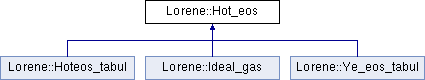## Public Member Functions

virtual ~Hot_eos ()
Destructor. More...

const string & get_name () const
Returns the hot EOS name. More...

void set_name (const char *)
Sets the hot EOS name. More...

virtual bool operator== (const Hot_eos &) const =0
Comparison operator (egality) More...

virtual bool operator!= (const Hot_eos &) const =0
Comparison operator (difference) More...

virtual int identify () const =0
Returns a number to identify the sub-classe of Hot_eos the object belongs to. More...

virtual void sauve (FILE *) const
Save in a file. More...

virtual const Eosnew_cold_Eos () const =0
Returns the corresponding cold Eos. More...

virtual double nbar_Hs_p (double ent, double sb) const =0
Computes the baryon density from the log-enthalpy and electronic fraction (virtual function implemented in the derived classes). More...

Scalar nbar_Hs (const Scalar &ent, const Scalar &sb, int nzet, int l_min=0) const
Computes the baryon density field from the log-enthalpy field and entropy per baryon. More...

virtual double ener_Hs_p (double ent, double sb) const =0
Computes the total energy density from the log-enthalpy and entropy per baryon (virtual function implemented in the derived classes). More...

Scalar ener_Hs (const Scalar &ent, const Scalar &sb, int nzet, int l_min=0) const
Computes the total energy density from the log-enthalpy and entropy per baryon. More...

virtual double press_Hs_p (double ent, double sb) const =0
Computes the pressure from the log-enthalpy and entropy per baryon (virtual function implemented in the derived classes). More...

Scalar press_Hs (const Scalar &ent, const Scalar &sb, int nzet, int l_min=0) const
Computes the pressure from the log-enthalpy and entropy per baryon. More...

virtual double temp_Hs_p (double ent, double sb) const =0
Computes the temperature from the log-enthalpy and entropy per baryon (virtual function implemented in the derived classes). More...

Scalar temp_Hs (const Scalar &ent, const Scalar &sb, int nzet, int l_min=0) const
Computes the temperature field from the log-enthalpy field and entropy per baryon. More...

virtual double csound_square_Hs_p (double ent, const double ye) const =0
Computes the sound speed squared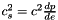from the enthapy with electronic fraction (virtual function implemented in the derived classes). More...

virtual double chi2_Hs_p (double ent, const double ye) const =0
Computes the chi^2 coefficient from the enthapy with electronic fraction (virtual function implemented in the derived classes). More...

Scalar chi2_Hs (const Scalar &ent, const Scalar &Ye, int nzet, int l_min=0) const
Computes the chi^2 coefficient from the enthalpy with ye. More...

virtual double mul_Hs_p (double ent, const double ye) const =0
Computes the electronic chemical potential from the enthapy with electronic fraction (virtual function implemented in the derived classes). More...

Scalar mul_Hs (const Scalar &ent, const Scalar &Ye, int nzet, int l_min=0) const
Computes the electronic chemical potential from the enthalpy with ye. More...

Scalar csound_square_Hs (const Scalar &ent, const Scalar &Y_e, int nzet, int l_min=0) const
Computes the sound speed squaredfrom the enthalpy with ye. More...

virtual double sigma_Hs_p (double ent, const double ye) const =0
Computes the source terms for electronic fraction advection equation from the enthapy with electronic fraction (virtual function implemented in the derived classes). More...

Scalar sigma_Hs (const Scalar &ent, const Scalar &Y_e, int nzet, int l_min=0) const
Computes the source terms for electronic fraction advection equation from the enthalpy with ye. More...

## Static Public Member Functions

static Hot_eoshoteos_from_file (FILE *)
Construction of an EOS from a binary file. More...

static Hot_eoshoteos_from_file (ifstream &)
Construction of a hot EOS from a formatted file. More...

## Protected Member Functions

Hot_eos ()
Standard constructor. More...

Hot_eos (const string &)
Standard constructor from a name (string) More...

Hot_eos (const char *)
Standard constructor from a name (char*) More...

Hot_eos (const Hot_eos &)
Copy constructor. More...

Hot_eos (FILE *)
Constructor from a binary file (created by the function sauve(FILE*) ). More...

Hot_eos (ifstream &)
Constructor from a formatted file. More...

virtual void del_deriv () const
Deletes all the derived quantities. More...

void set_der_0x0 () const
Sets to 0x0 all the pointers on derived quantities. More...

virtual ostream & operator>> (ostream &) const =0
Operator >> More...

void calcule (const Scalar &thermo1, const Scalar &thermo2, int nzet, int l_min, double(Hot_eos::*fait)(double, double) const, Scalar &resu) const
General computational method for Scalar 's. More...

## Protected Attributes

string name
EOS name. More...

Eosp_cold_eos
Corresponding cold Eos. More...

## Friends

ostream & operator<< (ostream &, const Hot_eos &)
Display. More...

## Detailed Description

Base class for 2-parameters equations of state (abstract class).

()

Definition at line 82 of file hoteos.h.

## ◆ Hot_eos() [1/6]

 Lorene::Hot_eos::Hot_eos ( )
protected

Standard constructor.

Definition at line 56 of file hoteos.C.

## ◆ Hot_eos() [2/6]

 Lorene::Hot_eos::Hot_eos ( const string & name_i )
explicitprotected

Standard constructor from a name (string)

Definition at line 60 of file hoteos.C.

References set_der_0x0().

## ◆ Hot_eos() [3/6]

 Lorene::Hot_eos::Hot_eos ( const char * name_i )
explicitprotected

Standard constructor from a name (char*)

Definition at line 64 of file hoteos.C.

References set_der_0x0().

## ◆ Hot_eos() [4/6]

 Lorene::Hot_eos::Hot_eos ( const Hot_eos & eos_i )
protected

Copy constructor.

Definition at line 70 of file hoteos.C.

References set_der_0x0().

## ◆ Hot_eos() [5/6]

 Lorene::Hot_eos::Hot_eos ( FILE * fich )
protected

Constructor from a binary file (created by the function sauve(FILE*) ).

This constructor is protected because any hot EOS construction from a binary file must be done via the function Hot_eos::hoteos_from_file(FILE*) .

Definition at line 76 of file hoteos.C.

References set_der_0x0(), and set_name().

## ◆ Hot_eos() [6/6]

 Lorene::Hot_eos::Hot_eos ( ifstream & fich )
protected

Constructor from a formatted file.

This constructor is protected because any hot EOS construction from a formatted file must be done via the function Hot_eos::hoteos_from_file(ifstream&) .

Definition at line 90 of file hoteos.C.

References set_der_0x0(), and set_name().

## ◆ ~Hot_eos()

 Lorene::Hot_eos::~Hot_eos ( )
virtual

Destructor.

Definition at line 101 of file hoteos.C.

References del_deriv().

## ◆ calcule()

 void Lorene::Hot_eos::calcule ( const Scalar & thermo1, const Scalar & thermo2, int nzet, int l_min, double(Hot_eos::*)(double, double) const fait, Scalar & resu ) const
protected

General computational method for Scalar 's.

Parameters
 thermo1 [input] first thermodynamical quantity (for instance the enthalpy field) from which the thermodynamical quantity resu is to be computed. thermo2 [input] second thermodynamical quantity (for instance the entropy field) from which the thermodynamical quantity resu is to be computed. nzet [input] number of domains where resu is to be computed. l_min [input] index of the innermost domain is which resu is to be computed [default value: 0]; resu is computed only in domains whose indices are in [l_min,l_min+nzet-1] . In the other domains, it is set to zero. fait [input] pointer on the member function of class Hot_eos which performs the pointwise calculation. resu [output] result of the computation.

Definition at line 153 of file hoteos.C.

References Lorene::Scalar::get_etat().

## ◆ chi2_Hs()

 Scalar Lorene::Hot_eos::chi2_Hs ( const Scalar & ent, const Scalar & Ye, int nzet, int l_min = 0 ) const

Computes the chi^2 coefficient from the enthalpy with ye.

Parameters
 ent [input, unit: c^2] enthalpy nzet number of domains where the derivative dln(e)/dln(H) is to be computed. l_min index of the innermost domain is which the coefficient dln(n)/dln(H) is to be computed [default value: 0]; the derivative dln(e)/dln(H) is computed only in domains whose indices are in [l_min,l_min+nzet-1] . In the other domains, it is set to zero. ye [input, unit: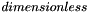] electronic fraction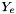Returns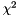[unit: c^2]

Definition at line 306 of file hoteos.C.

References calcule(), chi2_Hs_p(), and Lorene::Tensor::get_mp().

## ◆ chi2_Hs_p()

 virtual double Lorene::Hot_eos::chi2_Hs_p ( double ent, const double ye ) const
pure virtual

Computes the chi^2 coefficient from the enthapy with electronic fraction (virtual function implemented in the derived classes).

Parameters
 ent [input, unit: c^2] enthalpy ye [input, unit:] electronic fractionReturns[unit: c^2]

Implemented in Lorene::Ye_eos_tabul, Lorene::Hoteos_tabul, and Lorene::Ideal_gas.

## ◆ csound_square_Hs()

 Scalar Lorene::Hot_eos::csound_square_Hs ( const Scalar & ent, const Scalar & Y_e, int nzet, int l_min = 0 ) const

Computes the sound speed squaredfrom the enthalpy with ye.

Parameters
 ent [input, unit: c^2] enthalpy nzet number of domains where the derivative dln(e)/dln(H) is to be computed. l_min index of the innermost domain is which the coefficient dln(n)/dln(H) is to be computed [default value: 0]; the derivative dln(e)/dln(H) is computed only in domains whose indices are in [l_min,l_min+nzet-1] . In the other domains, it is set to zero. ye [input, unit:] electronic fractionReturns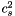[unit: c^2]

Definition at line 294 of file hoteos.C.

References calcule(), csound_square_Hs_p(), and Lorene::Tensor::get_mp().

## ◆ csound_square_Hs_p()

 virtual double Lorene::Hot_eos::csound_square_Hs_p ( double ent, const double ye ) const
pure virtual

Computes the sound speed squaredfrom the enthapy with electronic fraction (virtual function implemented in the derived classes).

Parameters
 ent [input, unit: c^2] enthalpy ye [input, unit:] electronic fractionReturns[unit: c^2]

Implemented in Lorene::Ye_eos_tabul, Lorene::Hoteos_tabul, and Lorene::Ideal_gas.

## ◆ del_deriv()

 void Lorene::Hot_eos::del_deriv ( ) const
protectedvirtual

Deletes all the derived quantities.

Definition at line 109 of file hoteos.C.

References p_cold_eos, and set_der_0x0().

## ◆ ener_Hs()

 Scalar Lorene::Hot_eos::ener_Hs ( const Scalar & ent, const Scalar & sb, int nzet, int l_min = 0 ) const

Computes the total energy density from the log-enthalpy and entropy per baryon.

Parameters
 ent [input, unit: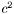] log-enthalpy H defined by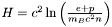, where e is the (total) energy density, p the pressure, n the baryon density, and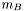the baryon mass sb [input, unit: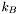] entropy per baryon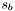nzet number of domains where the energy density is to be computed. l_min index of the innermost domain is which the energy density is to be computed [default value: 0]; the energy density is computed only in domains whose indices are in [l_min,l_min+nzet-1] . In the other domains, it is set to zero.
Returns
energy density [unit: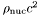], where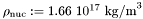Definition at line 252 of file hoteos.C.

References calcule(), ener_Hs_p(), and Lorene::Tensor::get_mp().

## ◆ ener_Hs_p()

 virtual double Lorene::Hot_eos::ener_Hs_p ( double ent, double sb ) const
pure virtual

Computes the total energy density from the log-enthalpy and entropy per baryon (virtual function implemented in the derived classes).

Parameters
 ent [input, unit:] log-enthalpy H defined by, where e is the (total) energy density, p the pressure, n the baryon density, andthe baryon mass sb [input, unit:] entropy per baryonReturns
energy density e [unit:], whereImplemented in Lorene::Ye_eos_tabul, Lorene::Hoteos_tabul, and Lorene::Ideal_gas.

## ◆ get_name()

 const string& Lorene::Hot_eos::get_name ( ) const
inline

Returns the hot EOS name.

Definition at line 135 of file hoteos.h.

References name.

## ◆ hoteos_from_file() [1/2]

 Hot_eos * Lorene::Hot_eos::hoteos_from_file ( FILE * fich )
static

Construction of an EOS from a binary file.

The file must have been created by the function sauve(FILE*) .

Definition at line 78 of file hoteos_from_file.C.

## ◆ hoteos_from_file() [2/2]

 Hot_eos * Lorene::Hot_eos::hoteos_from_file ( ifstream & fich )
static

Construction of a hot EOS from a formatted file.

The fist line of the file must start by the EOS number, according to the following conventions:

• 1 = relativistic ideal gas (class Ideal_gas ).
• 2 = non-relativistic ideal gas (class Ideal_gas_norel ).

The second line in the file should contain a name given by the user to the EOS. The following lines should contain the EOS parameters (one parameter per line), in the same order than in the class declaration.

Definition at line 120 of file hoteos_from_file.C.

## ◆ identify()

 virtual int Lorene::Hot_eos::identify ( ) const
pure virtual

Returns a number to identify the sub-classe of Hot_eos the object belongs to.

Implemented in Lorene::Ye_eos_tabul, Lorene::Hoteos_tabul, and Lorene::Ideal_gas.

## ◆ mul_Hs()

 Scalar Lorene::Hot_eos::mul_Hs ( const Scalar & ent, const Scalar & Ye, int nzet, int l_min = 0 ) const

Computes the electronic chemical potential from the enthalpy with ye.

Parameters
 ent [input, unit: c^2] enthalpy nzet number of domains where the derivative dln(e)/dln(H) is to be computed. l_min index of the innermost domain is which the coefficient dln(n)/dln(H) is to be computed [default value: 0]; the derivative dln(e)/dln(H) is computed only in domains whose indices are in [l_min,l_min+nzet-1] . In the other domains, it is set to zero. ye [input, unit:] electronic fractionReturns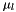[unit: m_n]

Definition at line 318 of file hoteos.C.

References calcule(), Lorene::Tensor::get_mp(), and mul_Hs_p().

## ◆ mul_Hs_p()

 virtual double Lorene::Hot_eos::mul_Hs_p ( double ent, const double ye ) const
pure virtual

Computes the electronic chemical potential from the enthapy with electronic fraction (virtual function implemented in the derived classes).

Parameters
 ent [input, unit: c^2] enthalpy ye [input, unit:] electronic fractionReturns[unit: m_n]

Implemented in Lorene::Ye_eos_tabul, Lorene::Hoteos_tabul, and Lorene::Ideal_gas.

## ◆ nbar_Hs()

 Scalar Lorene::Hot_eos::nbar_Hs ( const Scalar & ent, const Scalar & sb, int nzet, int l_min = 0 ) const

Computes the baryon density field from the log-enthalpy field and entropy per baryon.

Parameters
 ent [input, unit:] log-enthalpy H defined by, where e is the (total) energy density, p the pressure, n the baryon density, andthe baryon mass sb [input, unit:] entropy per baryonnzet number of domains where the baryon density is to be computed. l_min index of the innermost domain is which the baryon density is to be computed [default value: 0]; the baryon density is computed only in domains whose indices are in [l_min,l_min+nzet-1] . In the other domains, it is set to zero.
Returns
baryon density [unit: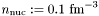]

Definition at line 236 of file hoteos.C.

References calcule(), Lorene::Tensor::get_mp(), and nbar_Hs_p().

## ◆ nbar_Hs_p()

 virtual double Lorene::Hot_eos::nbar_Hs_p ( double ent, double sb ) const
pure virtual

Computes the baryon density from the log-enthalpy and electronic fraction (virtual function implemented in the derived classes).

Parameters
 ent [input, unit:] log-enthalpy H defined by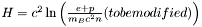, where e is the (total) energy density, p the pressure, n the baryon density, andthe baryon mass sb [input, unit:] electronic fractionReturns
baryon density [unit:]

Implemented in Lorene::Ye_eos_tabul, Lorene::Hoteos_tabul, and Lorene::Ideal_gas.

## ◆ new_cold_Eos()

 virtual const Eos& Lorene::Hot_eos::new_cold_Eos ( ) const
pure virtual

Returns the corresponding cold Eos.

Implemented in Lorene::Ye_eos_tabul, Lorene::Hoteos_tabul, and Lorene::Ideal_gas.

## ◆ operator!=()

 virtual bool Lorene::Hot_eos::operator!= ( const Hot_eos & ) const
pure virtual

Comparison operator (difference)

Implemented in Lorene::Ye_eos_tabul, Lorene::Hoteos_tabul, and Lorene::Ideal_gas.

## ◆ operator==()

 virtual bool Lorene::Hot_eos::operator== ( const Hot_eos & ) const
pure virtual

Comparison operator (egality)

Implemented in Lorene::Ye_eos_tabul, Lorene::Hoteos_tabul, and Lorene::Ideal_gas.

## ◆ operator>>()

 virtual ostream& Lorene::Hot_eos::operator>> ( ostream & ) const
protectedpure virtual

Operator >>

Implemented in Lorene::Ye_eos_tabul, Lorene::Hoteos_tabul, and Lorene::Ideal_gas.

## ◆ press_Hs()

 Scalar Lorene::Hot_eos::press_Hs ( const Scalar & ent, const Scalar & sb, int nzet, int l_min = 0 ) const

Computes the pressure from the log-enthalpy and entropy per baryon.

Parameters
 ent [input, unit:] log-enthalpy H defined by, where e is the (total) energy density, p the pressure, n the baryon density, andthe baryon mass sb [input, unit:] entropy per baryonnzet number of domains where the pressure is to be computed. l_min index of the innermost domain is which the pressure is to be computed [default value: 0]; the pressure is computed only in domains whose indices are in [l_min,l_min+nzet-1] . In the other domains, it is set to zero.
Returns
pressure [unit:], whereDefinition at line 266 of file hoteos.C.

References calcule(), Lorene::Tensor::get_mp(), and press_Hs_p().

## ◆ press_Hs_p()

 virtual double Lorene::Hot_eos::press_Hs_p ( double ent, double sb ) const
pure virtual

Computes the pressure from the log-enthalpy and entropy per baryon (virtual function implemented in the derived classes).

Parameters
 ent [input, unit:] log-enthalpy H defined by, where e is the (total) energy density, p the pressure, n the baryon density, andthe baryon mass sb [input, unit:] entropy per baryonReturns
pressure p [unit:], whereImplemented in Lorene::Ye_eos_tabul, Lorene::Hoteos_tabul, and Lorene::Ideal_gas.

## ◆ sauve()

 void Lorene::Hot_eos::sauve ( FILE * fich ) const
virtual

Save in a file.

Reimplemented in Lorene::Ye_eos_tabul, Lorene::Hoteos_tabul, and Lorene::Ideal_gas.

Definition at line 129 of file hoteos.C.

References Lorene::fwrite_be(), identify(), and name.

## ◆ set_der_0x0()

 void Lorene::Hot_eos::set_der_0x0 ( ) const
protected

Sets to 0x0 all the pointers on derived quantities.

Definition at line 114 of file hoteos.C.

References p_cold_eos.

## ◆ set_name()

 void Lorene::Hot_eos::set_name ( const char * name_i )

Sets the hot EOS name.

Definition at line 118 of file hoteos.C.

References name.

## ◆ sigma_Hs()

 Scalar Lorene::Hot_eos::sigma_Hs ( const Scalar & ent, const Scalar & Y_e, int nzet, int l_min = 0 ) const

Computes the source terms for electronic fraction advection equation from the enthalpy with ye.

Parameters
 ent [input, unit: c^2] enthalpy nzet number of domains where the derivative dln(e)/dln(H) is to be computed. l_min index of the innermost domain is which the coefficient dln(n)/dln(H) is to be computed [default value: 0]; the derivative dln(e)/dln(H) is computed only in domains whose indices are in [l_min,l_min+nzet-1] . In the other domains, it is set to zero. ye [input, unit:] electronic fractionReturns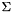[unit: 1/t_unit]

Definition at line 330 of file hoteos.C.

References calcule(), Lorene::Tensor::get_mp(), and sigma_Hs_p().

## ◆ sigma_Hs_p()

 virtual double Lorene::Hot_eos::sigma_Hs_p ( double ent, const double ye ) const
pure virtual

Computes the source terms for electronic fraction advection equation from the enthapy with electronic fraction (virtual function implemented in the derived classes).

Parameters
 ent [input, unit: c^2] enthalpy ye [input, unit:] electronic fractionReturns[unit: 1/t_unit]

Implemented in Lorene::Ye_eos_tabul, Lorene::Hoteos_tabul, and Lorene::Ideal_gas.

## ◆ temp_Hs()

 Scalar Lorene::Hot_eos::temp_Hs ( const Scalar & ent, const Scalar & sb, int nzet, int l_min = 0 ) const

Computes the temperature field from the log-enthalpy field and entropy per baryon.

Parameters
 ent [input, unit:] log-enthalpy H defined by, where e is the (total) energy density, p the pressure, n the baryon density, andthe baryon mass sb [input, unit:] entropy per baryonnzet number of domains where the baryon density is to be computed. l_min index of the innermost domain is which the baryon density is to be computed [default value: 0]; the baryon density is computed only in domains whose indices are in [l_min,l_min+nzet-1] . In the other domains, it is set to zero.
Returns
temperature [unit: MeV]

Definition at line 280 of file hoteos.C.

References calcule(), Lorene::Tensor::get_mp(), and temp_Hs_p().

## ◆ temp_Hs_p()

 virtual double Lorene::Hot_eos::temp_Hs_p ( double ent, double sb ) const
pure virtual

Computes the temperature from the log-enthalpy and entropy per baryon (virtual function implemented in the derived classes).

Parameters
 ent [input, unit:] log-enthalpy H defined by, where e is the (total) energy density, p the pressure, n the baryon density, andthe baryon mass sb [input, unit:] entropy per baryonReturns
temperature [unit: MeV]

Implemented in Lorene::Ye_eos_tabul, Lorene::Hoteos_tabul, and Lorene::Ideal_gas.

## ◆ operator<<

 ostream& operator<< ( ostream & ost, const Hot_eos & eqetat )
friend

Display.

Definition at line 143 of file hoteos.C.

## ◆ name

 string Lorene::Hot_eos::name
protected

EOS name.

Definition at line 87 of file hoteos.h.

## ◆ p_cold_eos

 Eos* Lorene::Hot_eos::p_cold_eos
mutableprotected

Corresponding cold Eos.

Definition at line 123 of file hoteos.h.

The documentation for this class was generated from the following files: